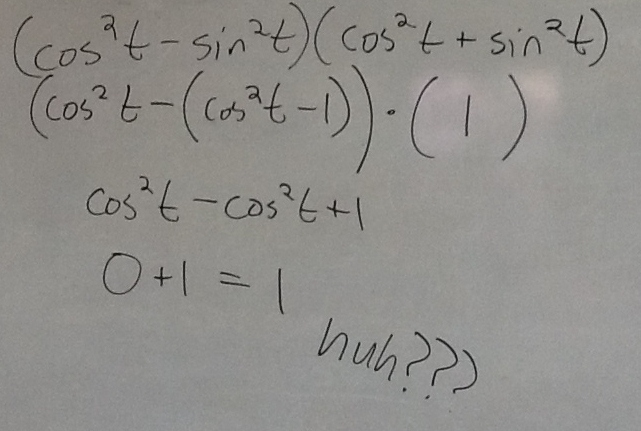Categories

# A Sneaky Simplifying MistakeThis is a fun mistake, via Jonathan Newman. Find it, and then explain how you’d help the kid who made it.

## 4 replies on “A Sneaky Simplifying Mistake”$sin^2(t) != cos^2(t) - 1$, it should be$sin^2(t) = 1 - cos^2(t)$. I think this is just a simple algebra mistake, or just going too quickly and not thinking through the subtraction properly.

Oh, looks like Latex in comments isn’t enabled.

Oh Isn’t Michael nice to fix our Latex? Let me give it a go ( my Sunday efforts!)$cos ^{2}t-( 1-cos ^{2}t) )$ which is$2( cos ^{2}t) -1$ or you can use the trig identity$cos 2t$ if you remember it.

Okay, Michael, punting to you. Again. It worked for cos 2t. the parentheses are wrong.$cos^(2)t -sin^(2)t$ which is$2(cos^(2)t -1$ or you can use the trig identity$cos2t$ if you remember it.